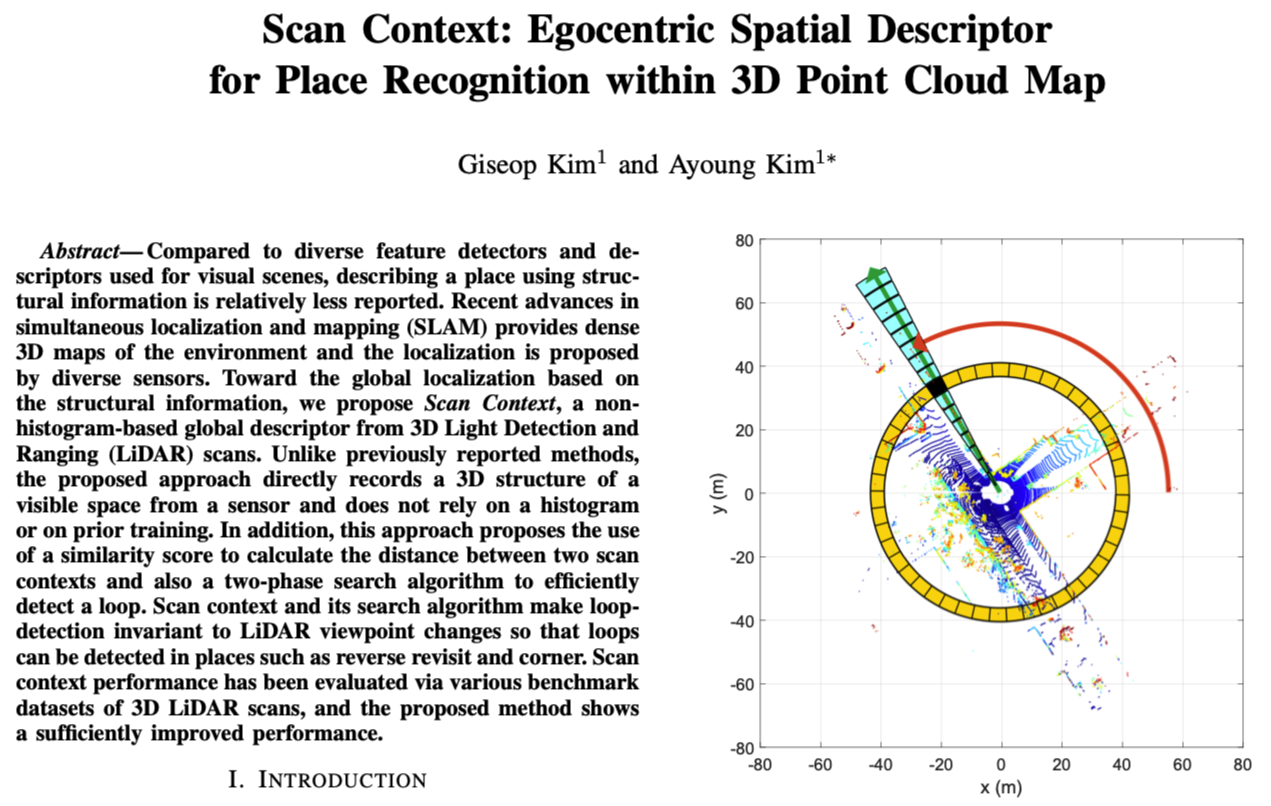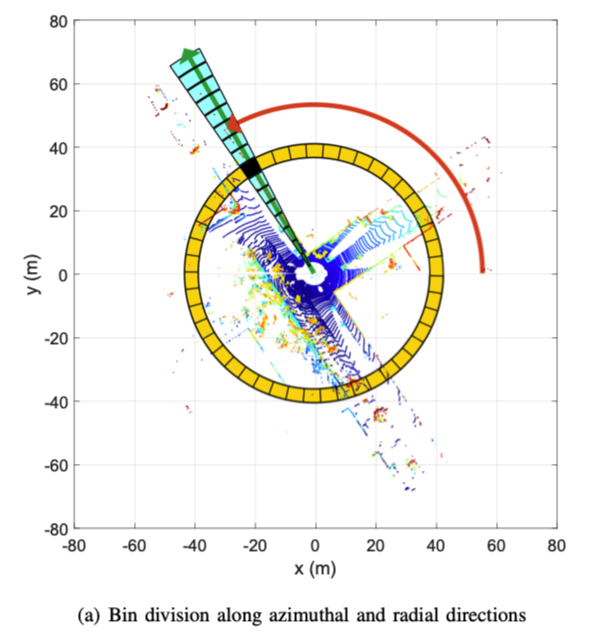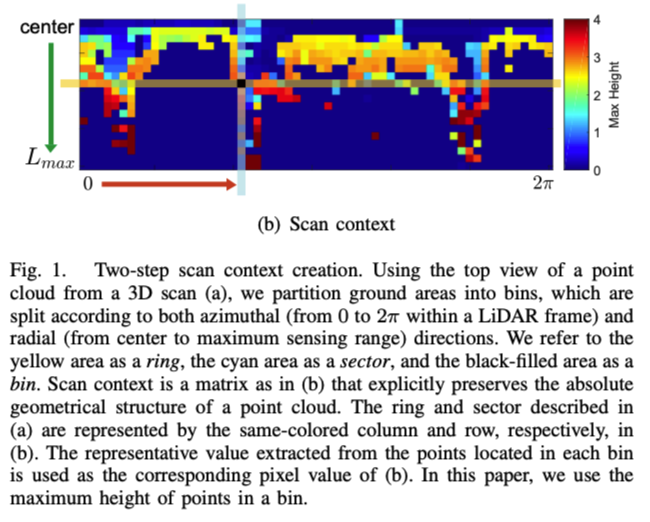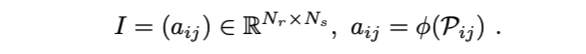# Scan Context: Egocentric Spatial Descriptor for Place Recognition within 3D Point Cloud Map# 介绍

• 无论视点如何变化，描述符需要有旋转不变性
• 噪声处理，因为点云的分辨率随距离变化，而且法线受噪声影响

• 高效的bin编码函数。与现有的点云描述符[7，10]不同，所提出的方法不需要计算容器中的点数，而是提出了一种更有效的容器编码功能来进行位置识别，此编码对点云密度和法线具有不变性
• 保存点云内部结构。如图1所示，矩阵的每个元素值仅由属于bin的点云确定，因此，与不同，后者将点的相对几何形状描述为直方图，并且会丢失点的绝对位置信息。我们的方法有意避免使用直方图来保留点云的绝对内部结构。这有利于提高判别能力，当距离得到后，还允许使得查询扫描帧与候选扫描视点对齐（实验中使用6$$\degree$$分辨率），因此本方法可用于检测反向的loop
• 高效的二阶段匹配算法。为了获得可行的搜索时间，我们为第一个最近邻搜索提供了一个旋转不变子描述符，并将其与成对相似性评分进行分层组合

# Scan Context• $$N_s$$ 扇形的数量（图a蓝色块数量）
• $$N_r$$ 环的数量 （图a红色块数量）
• $$\frac{L_{max}}{N_r}$$ 放射间隔，其中$$L_{max}$$表示激光最远距离
• $$\frac{2\pi}{N_s}$$ 扇形中心角
• 文章采用: $$N_s=60,N_r=20$$# 计算Scan Contexts的相似得分